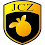## Thursday 19 November 2009

### ggplot2 is awesome

I like R, but R+ggplot2 is awesome.`library("ggplot2")x = seq(-2*pi, 2*pi, by = 0.05)x1 = x + rnorm(length(x))/10qplot(x, sin(x1), color=rgb(abs(sin(x)),0,1), geom = c("point", "smooth"))`

Requires that you `install.packages()` ggplot2, of course.

I'm currently working through Getting started with qplot (pdf).

#### 1 comment:

1.Industrial laser engraver will enlarge the horizon is no longer set in these single areas of developmen